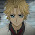## Sunday, April 15, 2012

### General Eigen Values in OpenCV

Calculating Generalized Eigen Values and Eigen Vectors in one of the very trivial tasks in languages like Matlab, Python(Numpy) in scientific computing. However, I've observed that finding Generalized Eigen Values, Vectors is one of the most common questions asked in regards with OpenCV. When you are doing something like LDA dimensionlaity reduction, you will need general Eigen Values, Vectors. This is useful in many other cases which need general eigen values to solve problems in OpenCV.

Calculating them in OpenCV is not a straight forward task.

Btw, My other programs in OpenCV will be posted here.

Also, calculated Eigen Values, Vectors are not sorted according to the correspoding Eigen Values. I've written down a solution to do this in OpenCV.

My solution uses Eigen3 library. It is a 3rd party library compatible with OpenCV 2.2+ (It does inear algebra). If you do not have Eigen3 Library, you can install on your debian system from the following
link or do execute following command:

Now that Eigen3 library is installed, you can use a cv::Mat(of whose you would want to calculate general Eigen Values and Vectors) and convert it to Matrix in Eigen3(to solve General Eigen Value problem in Eigen3 library) and then convert it back to cv::Mat.

Also the following code sorts the eigen values and vectors according to descending order which is what Matlab, Python(Numpy) do by default (while calculating Eigen Values, Vectors).

Matlab does it the following way:
Numpy-Scipy(Python) does it the following way:

Now doing the same in OpenCV 2.3(C++) using Eigen3 library:
** CODE UPDATED**
If you find any bug in the above code, please let me know.

1.2.